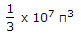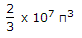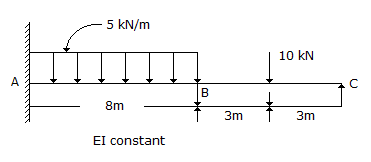# Civil Engineering - UPSC Civil Service Exam Questions

1.

A bar 4 cm in diameter is subjected to an axial load of 41. The extension of the bar over a gauge length of 20 cm is 0.03 cm. The decrease in diameter is 0.0018 cm. The Poisson's ratio is

 A. 0.25 B. 0.3 C. 0.33 D. 0.35

Explanation:

No answer description available for this question. Let us discuss.

2.

The kinetic energy in Nm of a circular disc of 20 m diameter and 10 cm thick, of material weighing 100 kg/m3, rotating above an axis through its centre at right angles to its place and making 2000 rpm is

 A.B.C. 2 x 108 π3 D. 4 x 108 π3

Explanation:

No answer description available for this question. Let us discuss.

3.

The upper limit of area ratio for which the amount of disturbance of soil sample can be considered to be small is

 A. 10% B. 15% C. 20% D. 25%

Explanation:

No answer description available for this question. Let us discuss.

4.

For the beam shown in figure, what are the distribution factors at joint B ?A. 0.4, 0.6 B. 0.5, 0.5 C. 0.6, 0.4 D. 0.65, 0.35

Explanation:

No answer description available for this question. Let us discuss.

5.

Consider the following statements :
The daily per capita consumption of water apparently increases with
1. higher standard of living of people.
2. availability of sewerage in the city.
3. metered water supply.
4. wholesome and potable quality of water.
Which of these statements are correct ?

 A. 1, 2 and 3 B. 2, 3 and 4 C. 1, 3 and 4 D. 1, 2 and 4